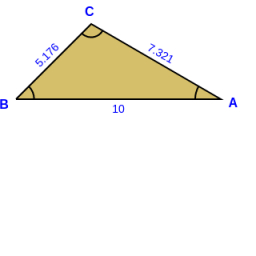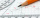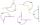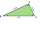# The two

The two angles of a triangle are 78° and 82°. So what is the measure of the remaining third angle?

C =  20 °

### Step-by-step explanation:Did you find an error or inaccuracy? Feel free to write us. Thank you!Tips to related online calculators

#### You need to know the following knowledge to solve this word math problem:

We encourage you to watch this tutorial video on this math problem:

## Related math problems and questions:

• TrianglesFind out whether given sizes of the angles can be interior angles of a triangle: a) 23°10',84°30',72°20' b) 90°,41°33',48°37' c) 14°51',90°,75°49' d) 58°58',59°59',60°3'
• A straight angleA straight angle measures s, so you can subtract or add 85 from What are the measure of their angles?
• Complementary anglesTwo angles are complementary. If one angle measures 10°, what is the measure of the second angle?
• The sum 11The sum of the measures of two angles is 89°. One angle has a measure of 64°. What is the measure in degrees of the second angle?
• AnglesThe triangle is one outer angle 158°54' and one internal angle 148°. Calculate the other internal angles of a triangle.
• 3-bracket 3Two angles in a triangle are 90° and 60°. Has triangle at least two equal sides?
• The angles ratioThe angles in the ABC triangle are in the ratio 1: 2: 3. find the sizes of the angles and determine what kind of a triangle it is.
• Outer anglesThe outer angle of the triangle ABC at the A vertex is 71°40 ' outer angle at the vertex B is 136°50'. What size has the inner triangle angle at the vertex C?
• Inner anglesThe magnitude of the internal angle at the main vertex C of the isosceles triangle ABC is 72°. The line p, parallel to the base of this triangle, divides the triangle into a trapezoid and a smaller triangle. How big are the inner angles of the trapezoid?
• Gamma angleFind the magnitude of the gamma angle in triangle ABC if: α = 38° 56 ’and β = 47° 54’.
• Two anglesThe triangles ABC and A'B'C 'are similar. In the ABC triangle, the two angles are 25° and 65°. Explain why in the triangle A'B'C 'is the sum of two angles of 90 degrees.
• SimilarityAre two right triangles similar to each other if the first one has an acute angle 70°, and the second one has an acute angle 20°?
• The trianglesThe triangles ABC and A'B'C 'are similar with a similarity coefficient of 2. The angles of the triangle ABC are alpha = 35°, beta = 48°. Determine the magnitudes of all angles of triangle A'B'C '.
• Internal and external anglesCalculate the remaining internal and external angles of a triangle, if you know the internal angle γ (gamma) = 34 degrees and one external angle is 78 degrees and 40 '. Determine what kind of triangle it is from the size of its angles.
• Find theFind the third interior angle of the triangle ABC where: α = 48°, γ = 65°.
• RWYCalculate the opposite direction of the runway 13. Runways are named by a number between 01 and 36, which is generally one tenth of the azimuth of the runway's heading in degrees: a runway numbered 09 points east (90°), runway 18 is south (180°), runway 2
• Length of the arcWhat is the arc length of a circle k (S, r=68mm), which belongs to a central angle of 78°?